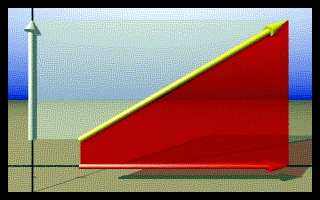# The Physics Department - Mechanics, VectorsAn understanding of vectors is essential for an understanding of physics. A vector is a quantity that has two aspects. It has a size, or magnitude, and a direction. In contrast, there a quantities called scalars that have only size.

Vectors are usually drawn as arrows. In the picture above, a two dimensional (2D) vector is drawn in yellow. This vector has two parts, or components. Its x-component, drawn in red, is positioned as if it were a shadow of the yellow vector on the x-axis. The white vector, positioned as a shadow on the y-axis, is the y-component of the yellow vector.

Custom Search